15. interface and application programming¶

MIT app inventor¶

For this weeks assigment i want to try MIT app inventor since it is the firt time i used this I started with a tuturial i found online before making something of my own.

tutorial¶

I wnated to make an app to change the colour of a RGB led because mayby I can implemet this in my final project. I followed this tutorial

setting up the app enviroment¶

IT works very user friendly you can click draw all the components to the right place.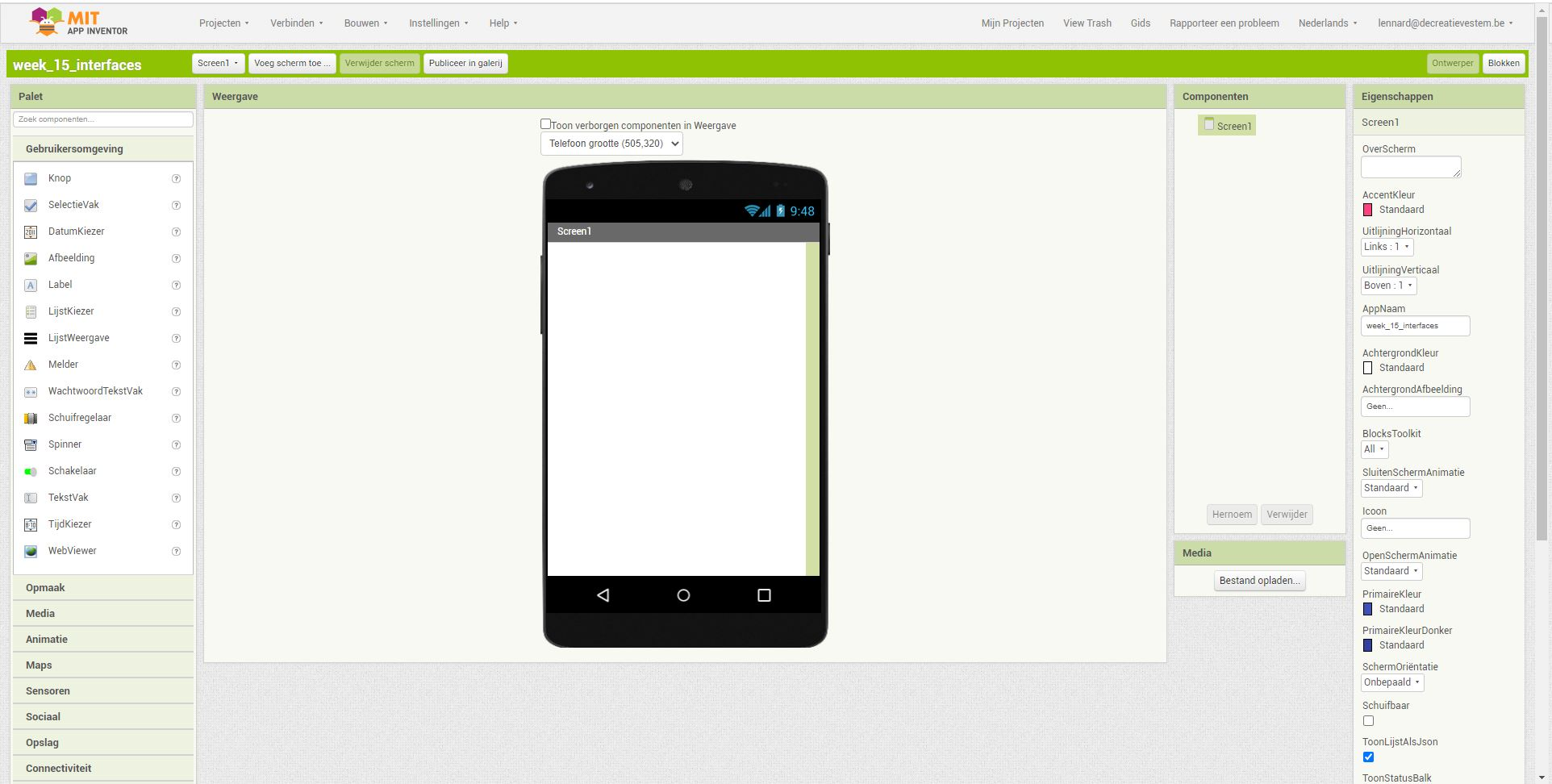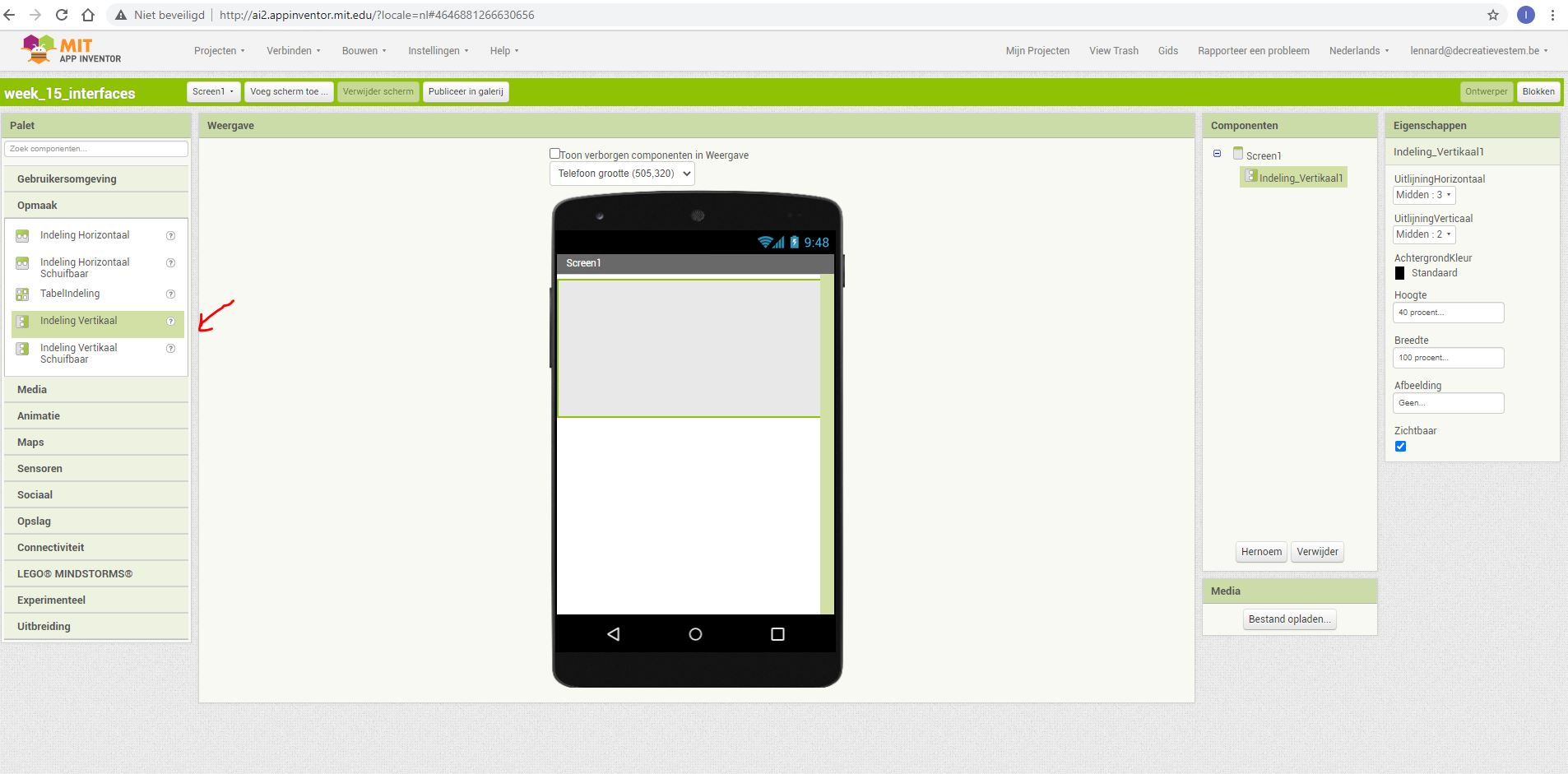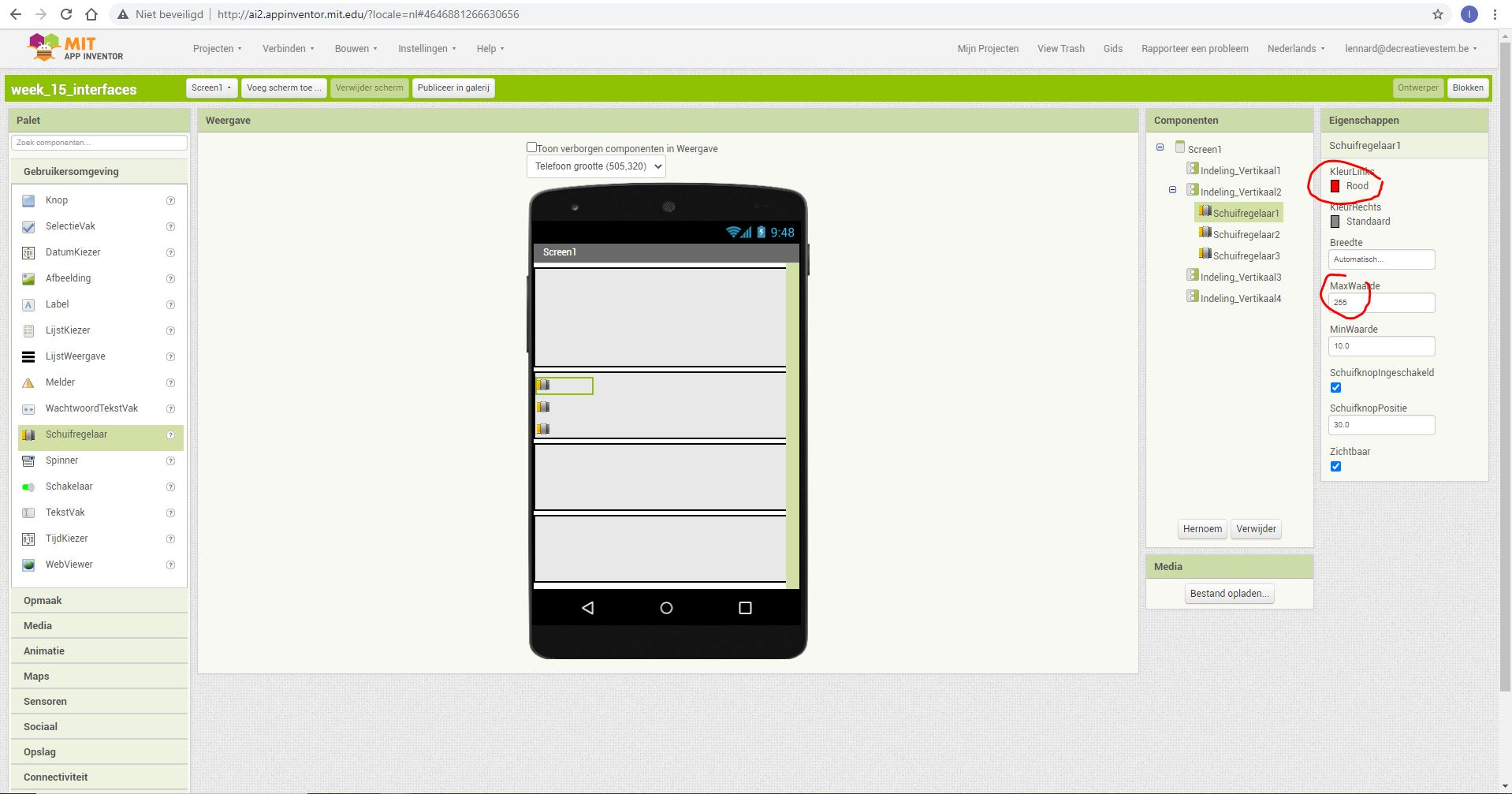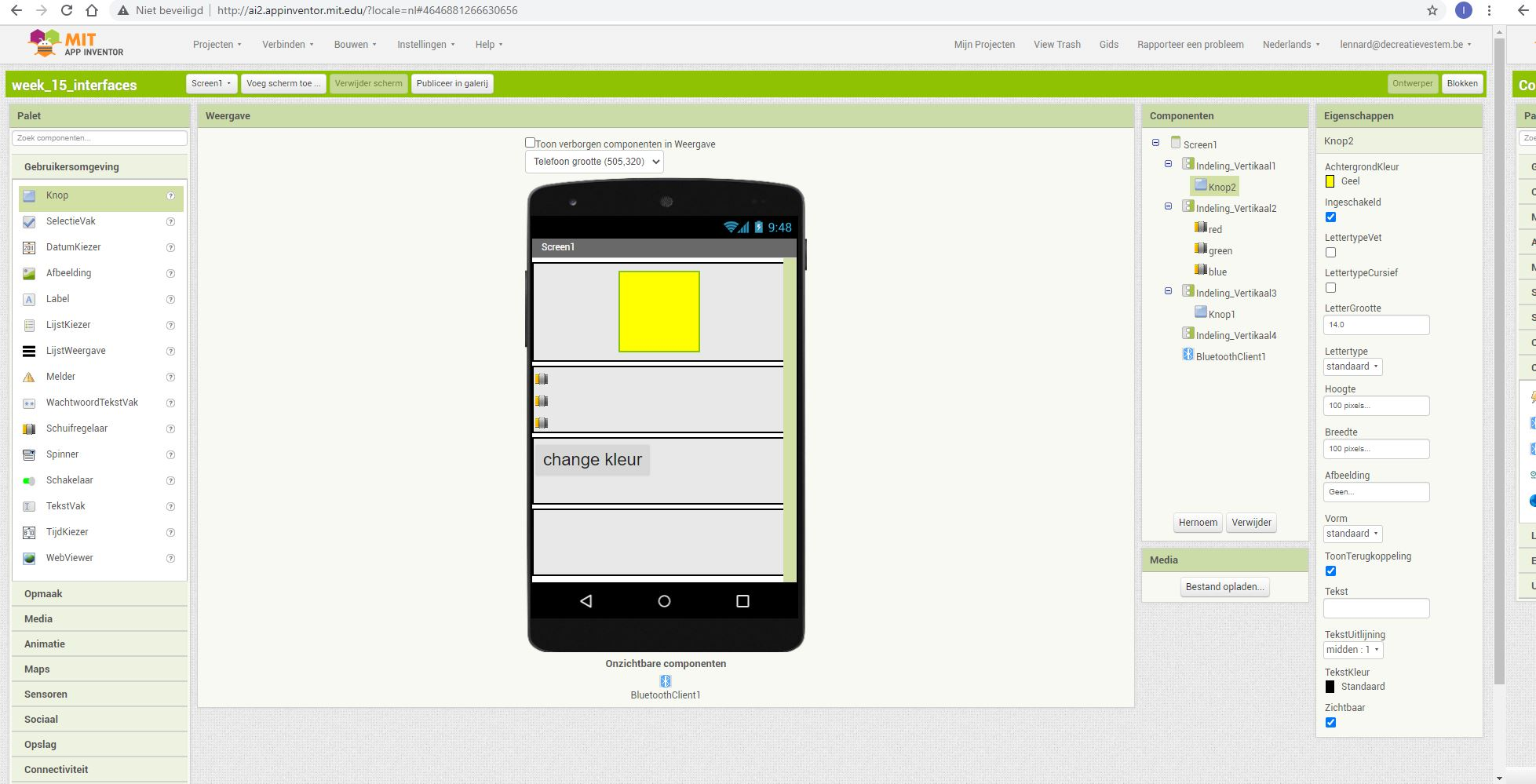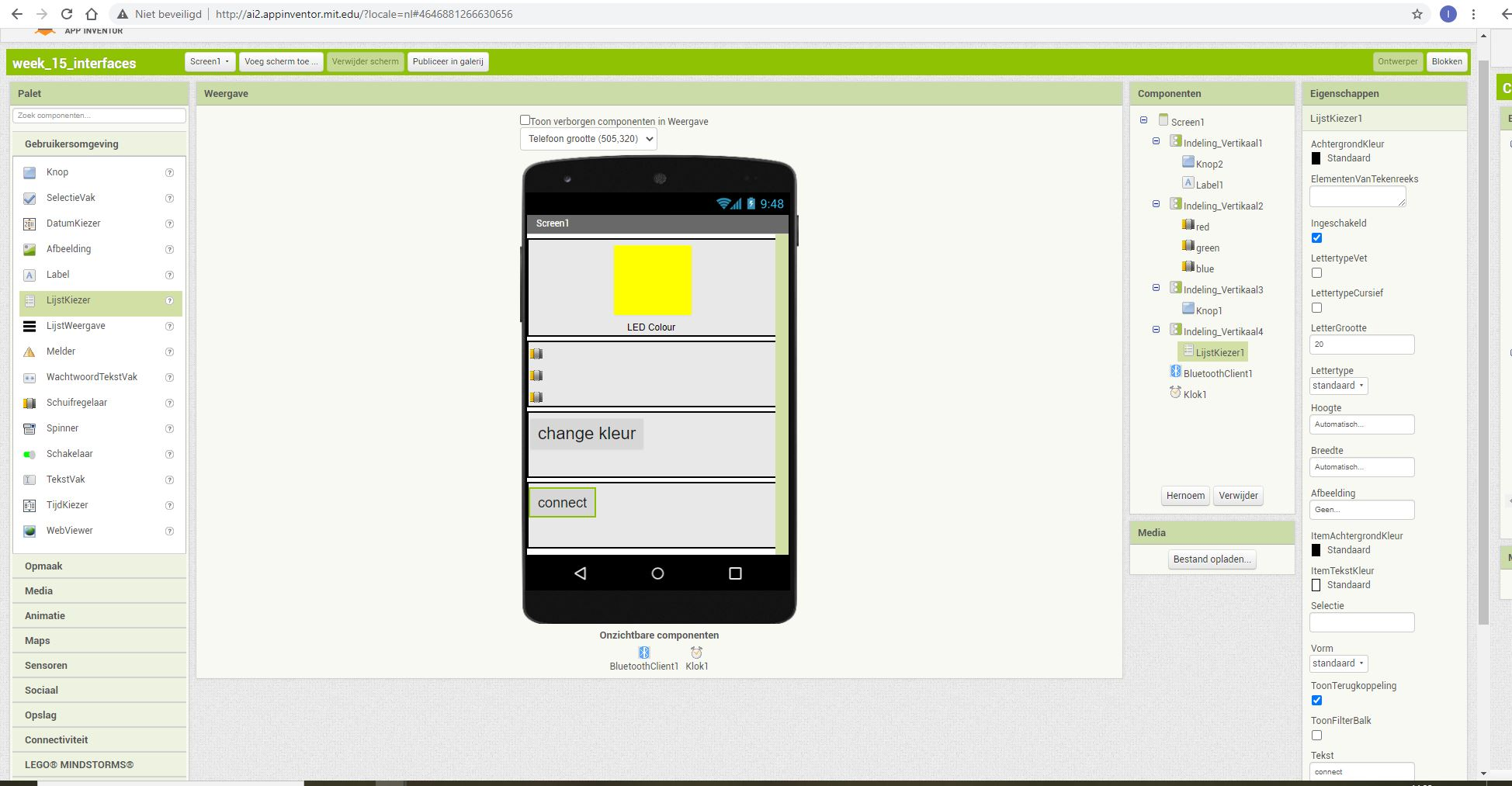When the enviroment is ready i started with the code behind everything.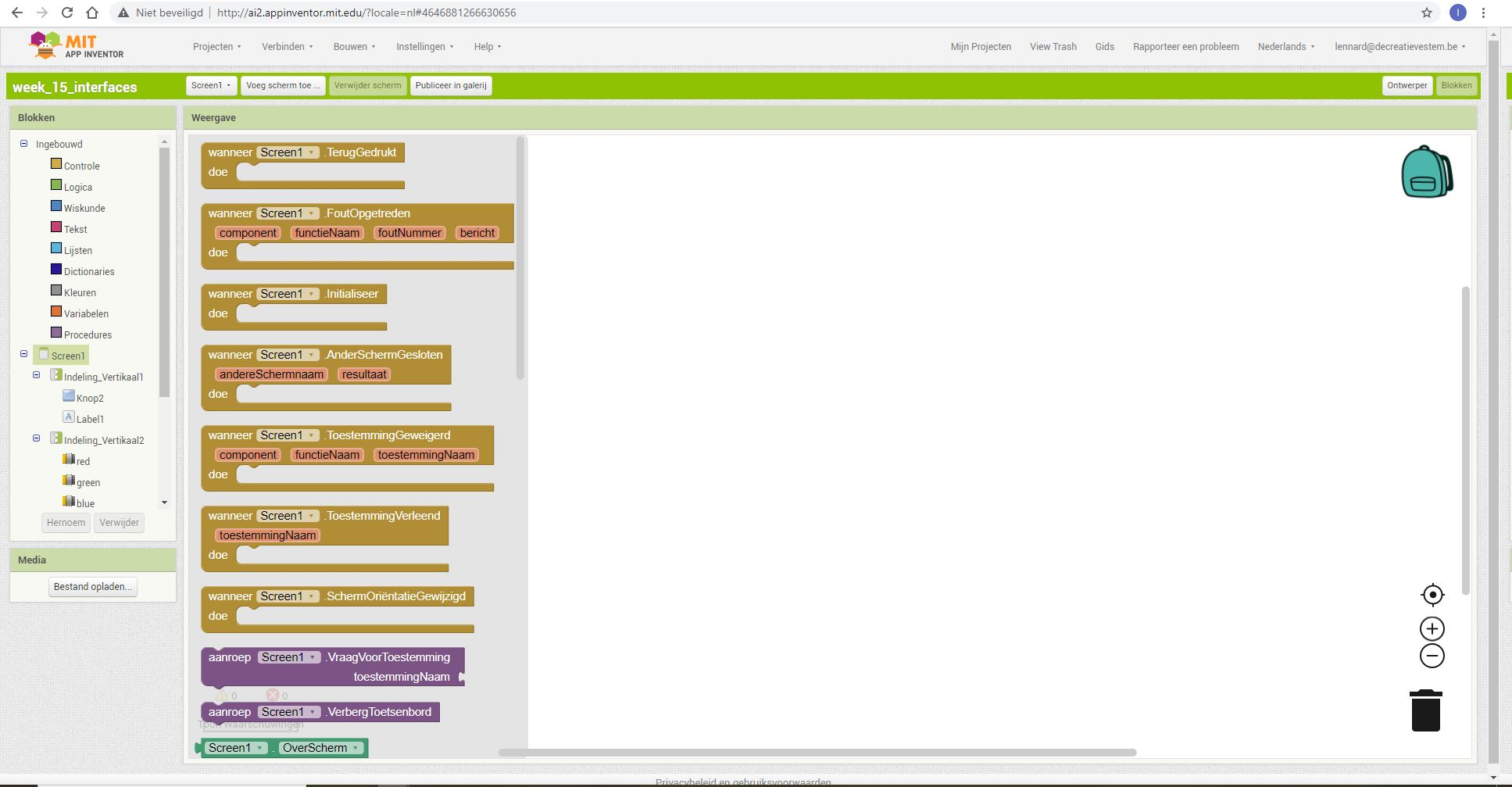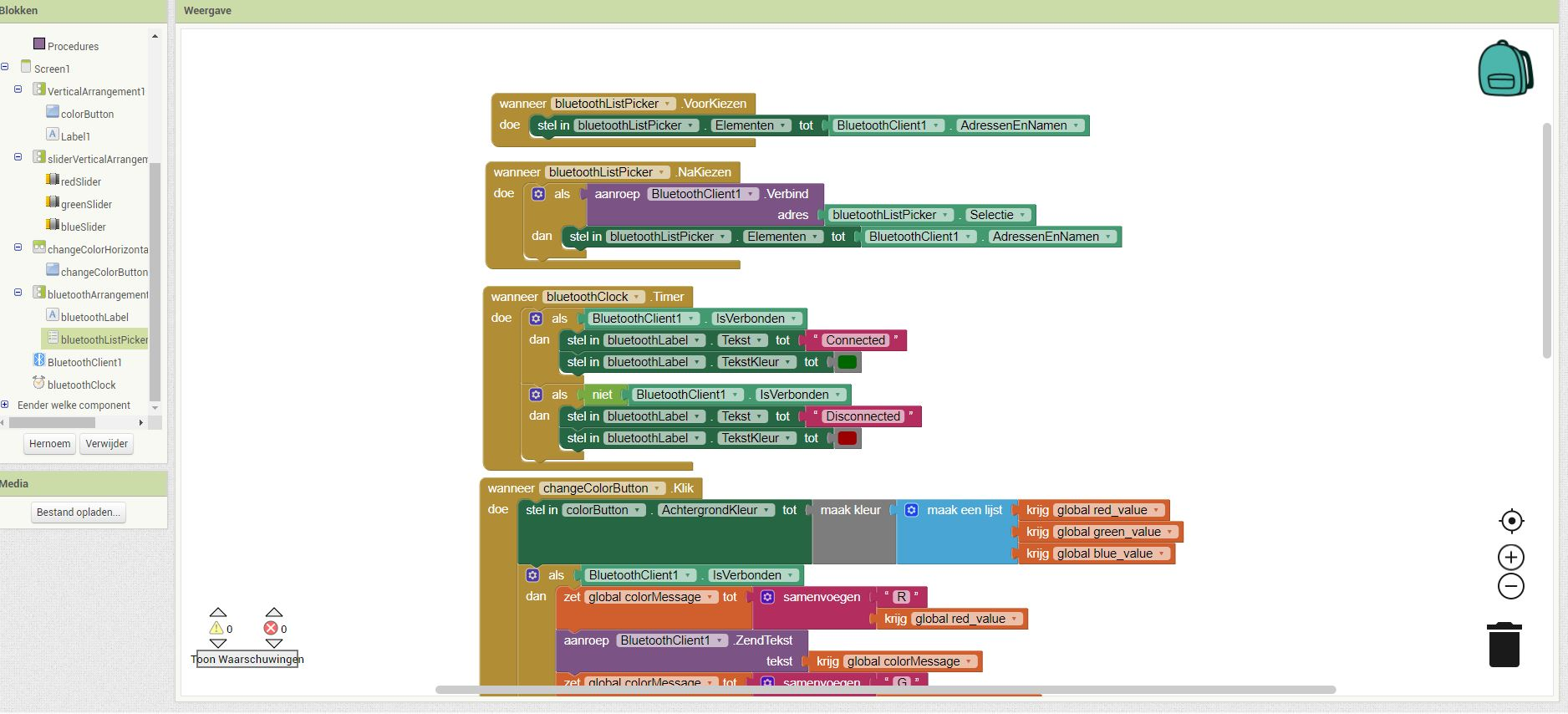building the electronic part¶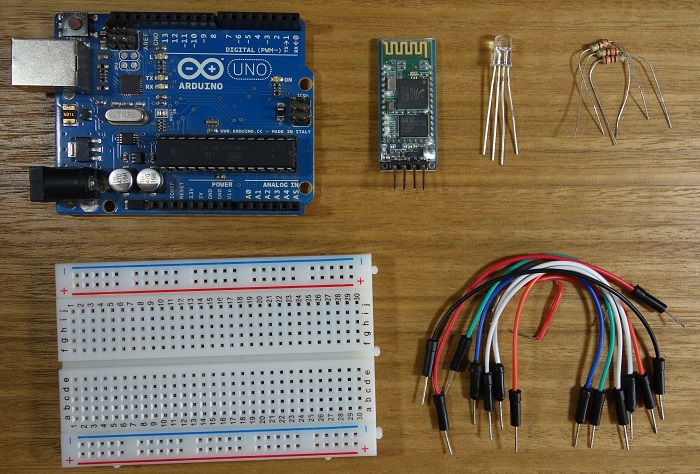• Arduino UNO
• Bluetooth module HC-05
• RGB LED
• 3 x 220Ohm resistor
• Jumper wires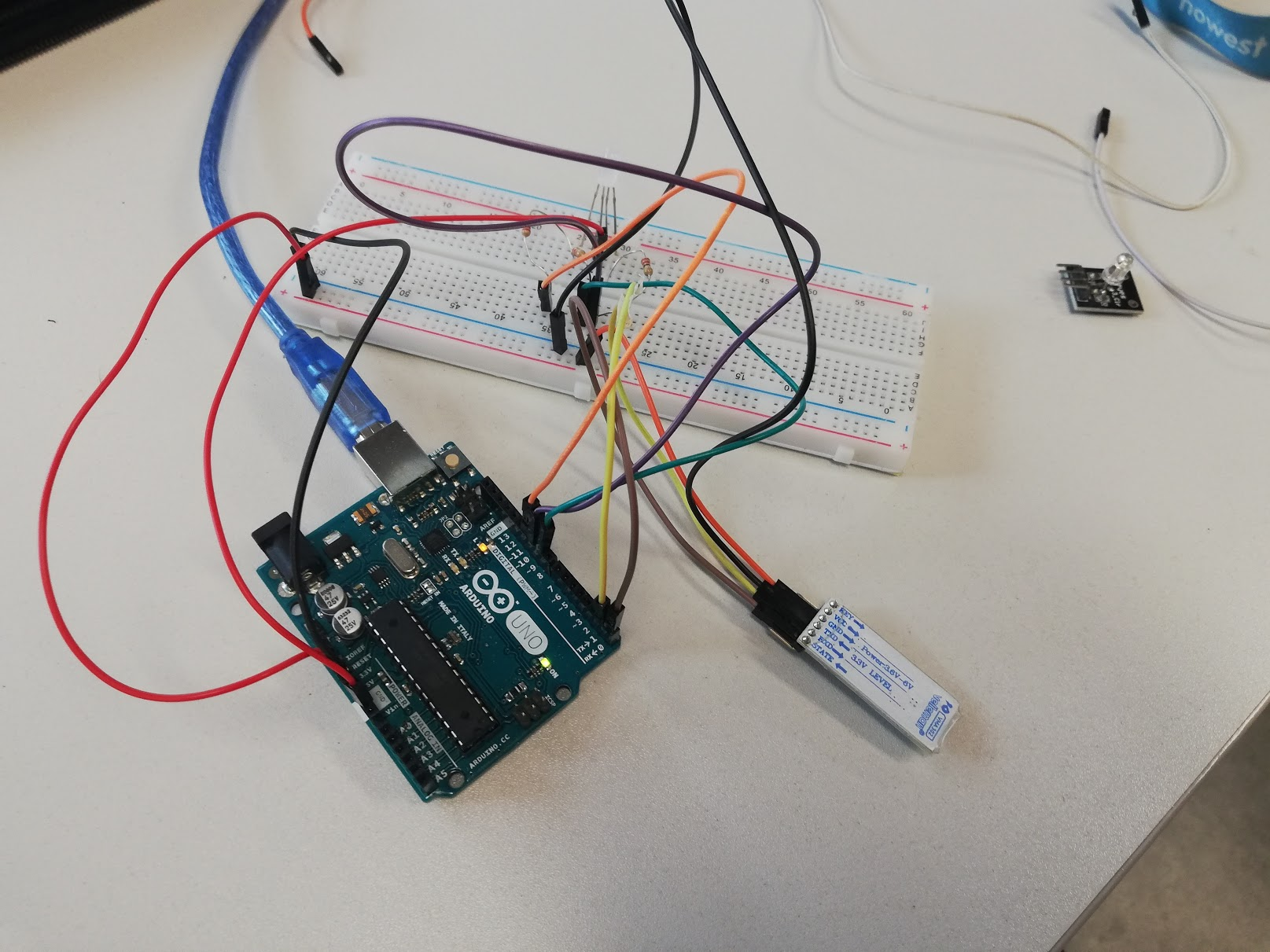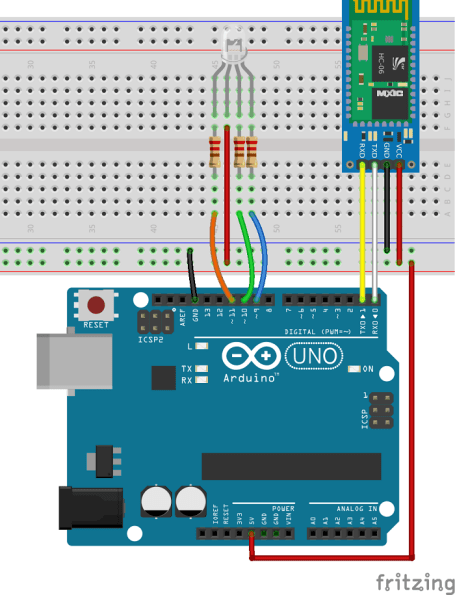installing the app on the phone¶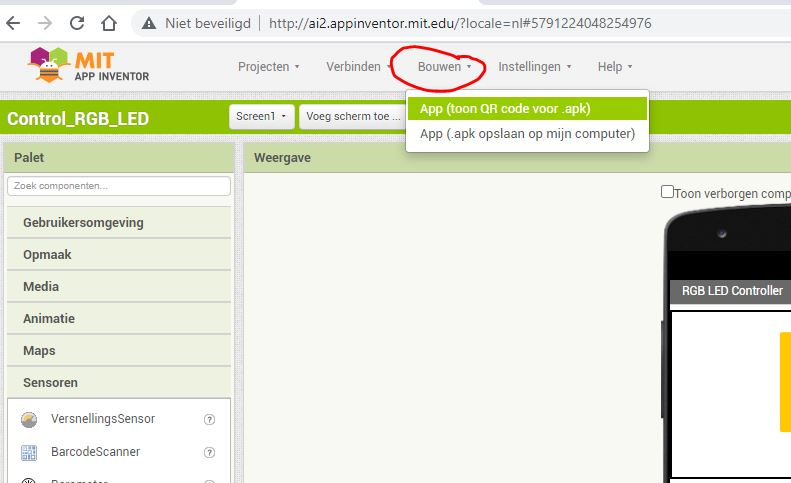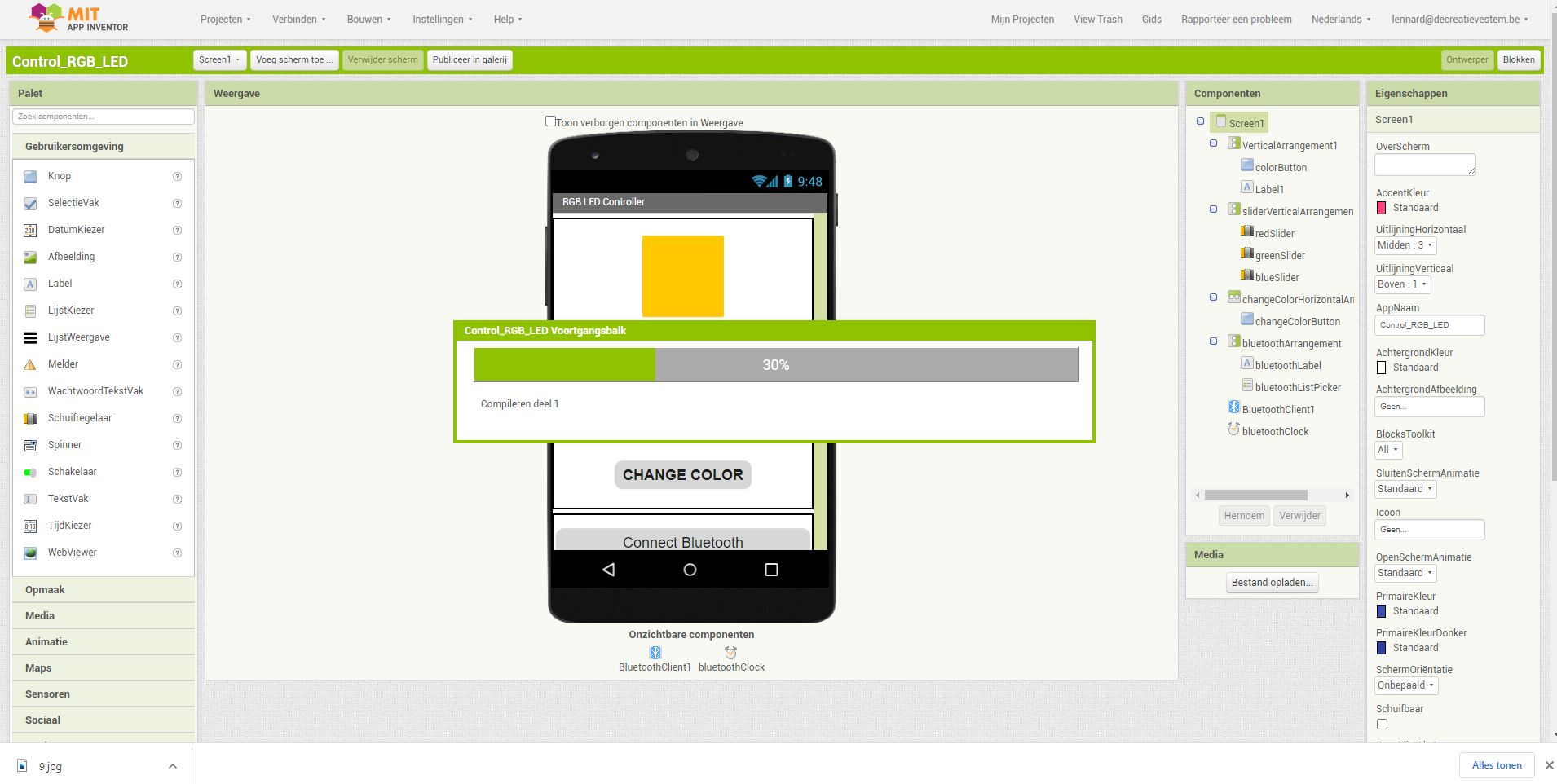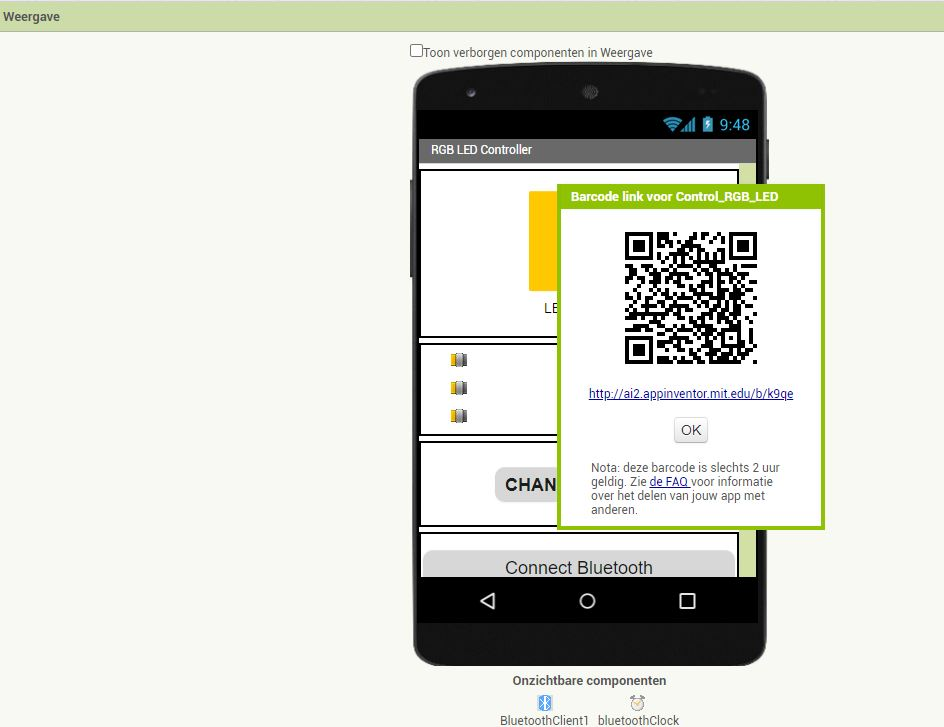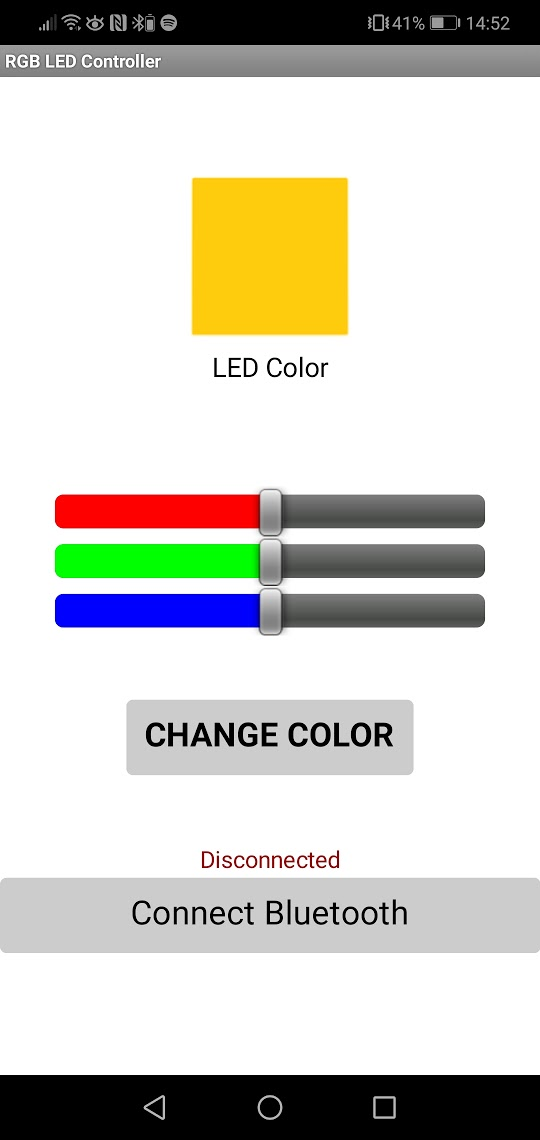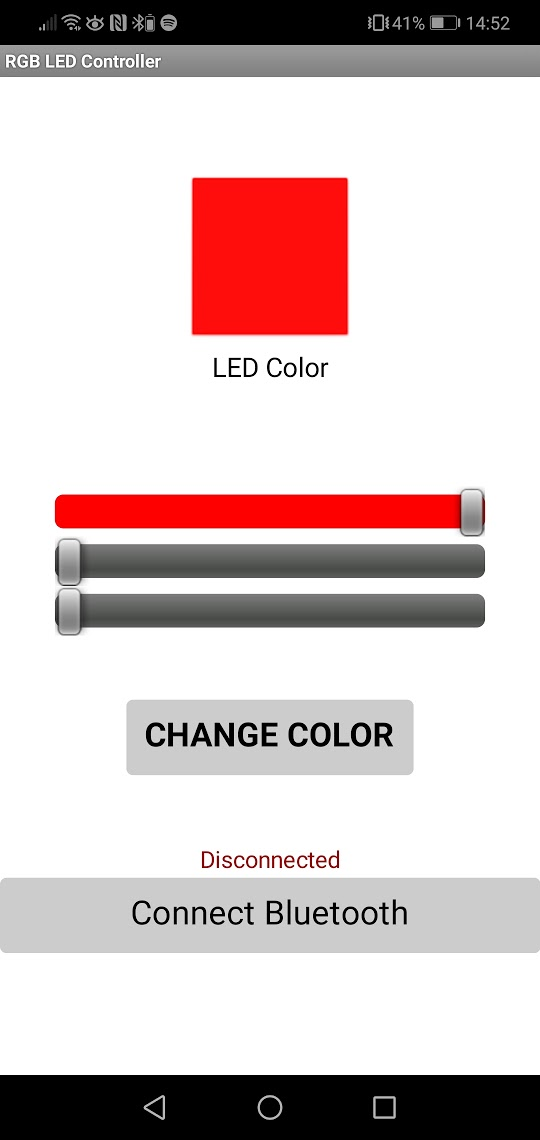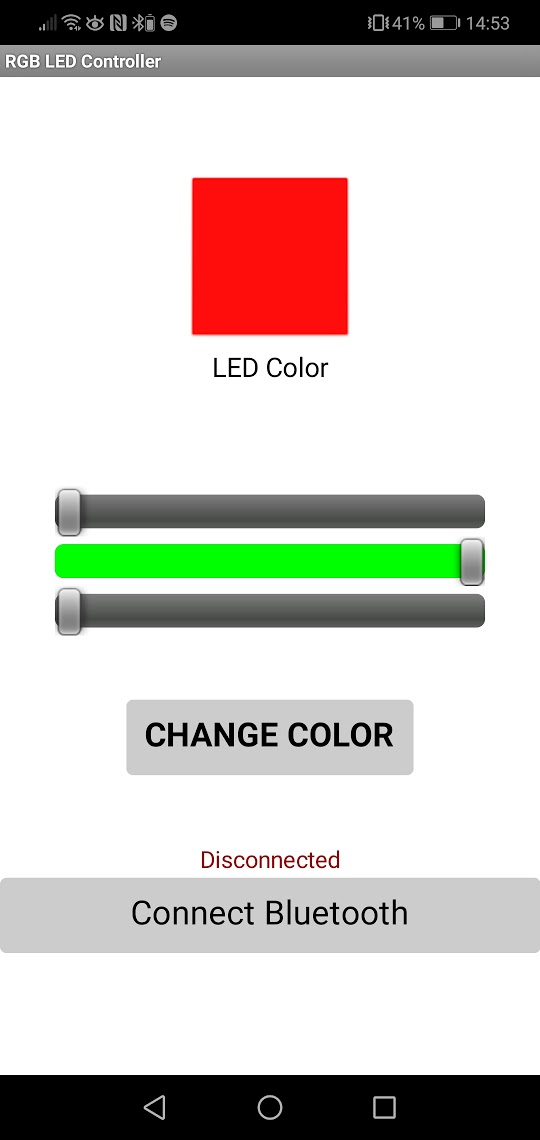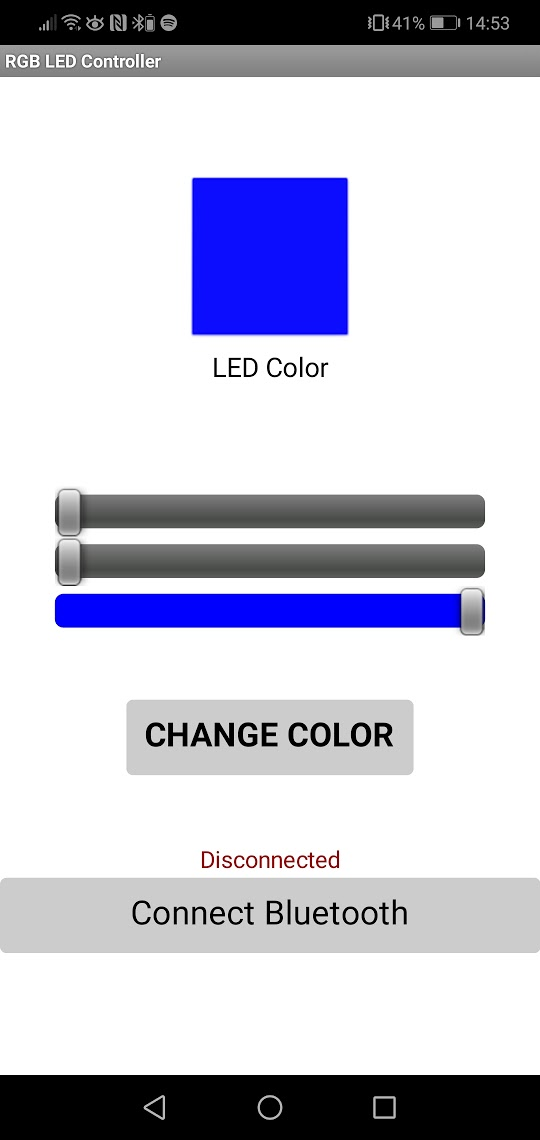could connect with the bluethooth module on the bradboard and could change the colour af the led therefore I had the program the arduino with the following code i got from the instructable.

/*
* Rui Santos
* Complete Project Details https://randomnerdtutorials.com
*/

#define max_char 12
char message[max_char];    // stores you message
char r_char;               // reads each character
byte index = 0;            // defines the position into your array
int i;

int redPin = 11;     // Red RGB pin -> D11
int greenPin = 10;   // Green RGB pin -> D10
int bluePin = 9;     // Blue RGB pin -> D9

int redValue = 255;     // Red RGB pin -> D11
int greenValue = 255;   // Green RGB pin -> D10
int blueValue = 255;     // Blue RGB pin -> D9

String redTempValue;     // Red RGB pin -> D11
String greenTempValue;   // Green RGB pin -> D10
String blueTempValue;     // Blue RGB pin -> D9

int flag = 0;
char currentColor;

void setup() {
pinMode(redPin,OUTPUT);
pinMode(bluePin,OUTPUT);
pinMode(greenPin, OUTPUT);
// initialize serial communication at 9600 bits per second:
Serial.begin(9600);
}

void loop() {
while(Serial.available() > 0){
flag = 0;
//the message can have up to 12 characters
if(index < (max_char-1)){
message[index] = r_char;     // Stores the character in message array
if(r_char=='R'){
currentColor = 'R';
redTempValue = "";
}
else if(r_char=='G'){
currentColor = 'G';
greenTempValue = "";
}
else if(r_char=='B'){
currentColor = 'B';
blueTempValue = "";
}
if(currentColor == 'R' && r_char!='R'){
redTempValue += r_char;
}
else if(currentColor == 'G' && r_char!='G'){
greenTempValue += r_char;
}
else if(currentColor == 'B' && r_char!='B'){
blueTempValue += r_char;
}
index++;                     // Increment position
message[index] = '\0';       // Delete the last position
}

}

if(flag == 0){
analogWrite(redPin, 255-redTempValue.toInt());
analogWrite(greenPin, 255-greenTempValue.toInt());
analogWrite(bluePin, 255-blueTempValue.toInt());
/*Serial.print('R');
Serial.println(redTempValue);
Serial.print('G');
Serial.println(greenTempValue);
Serial.print('B');
Serial.println(blueTempValue);
Serial.print("MESSAGE ");*/
Serial.println(message);
flag=1;
for(i=0; i<12; i++){
message[i] = '\0';
}
//resests the index
index=0;
}

}

making a game.¶

After testing the first tutorial I followed an extra lessons in the lab about app inventor. The teacher helped me with making the game wack-a-moll.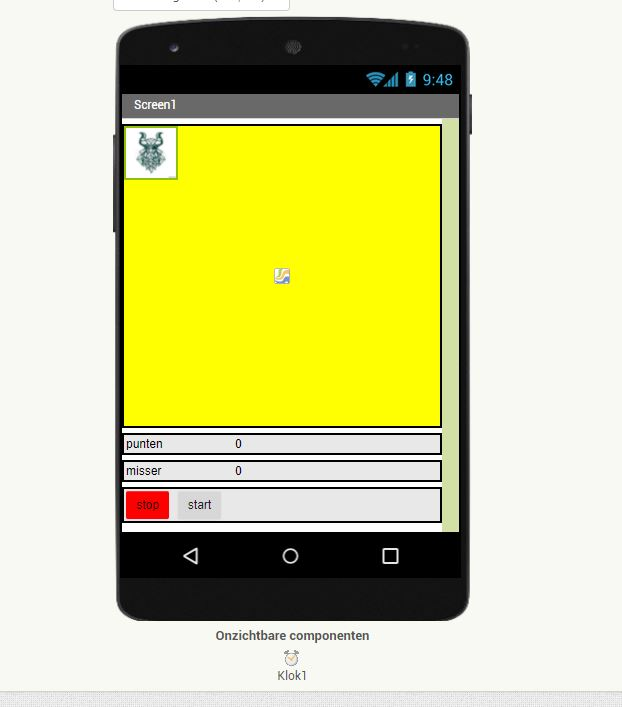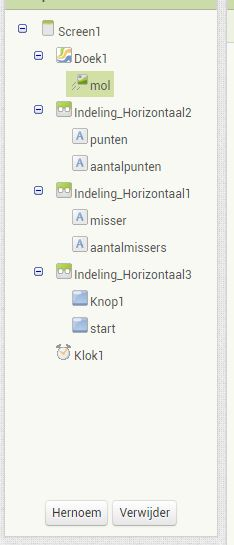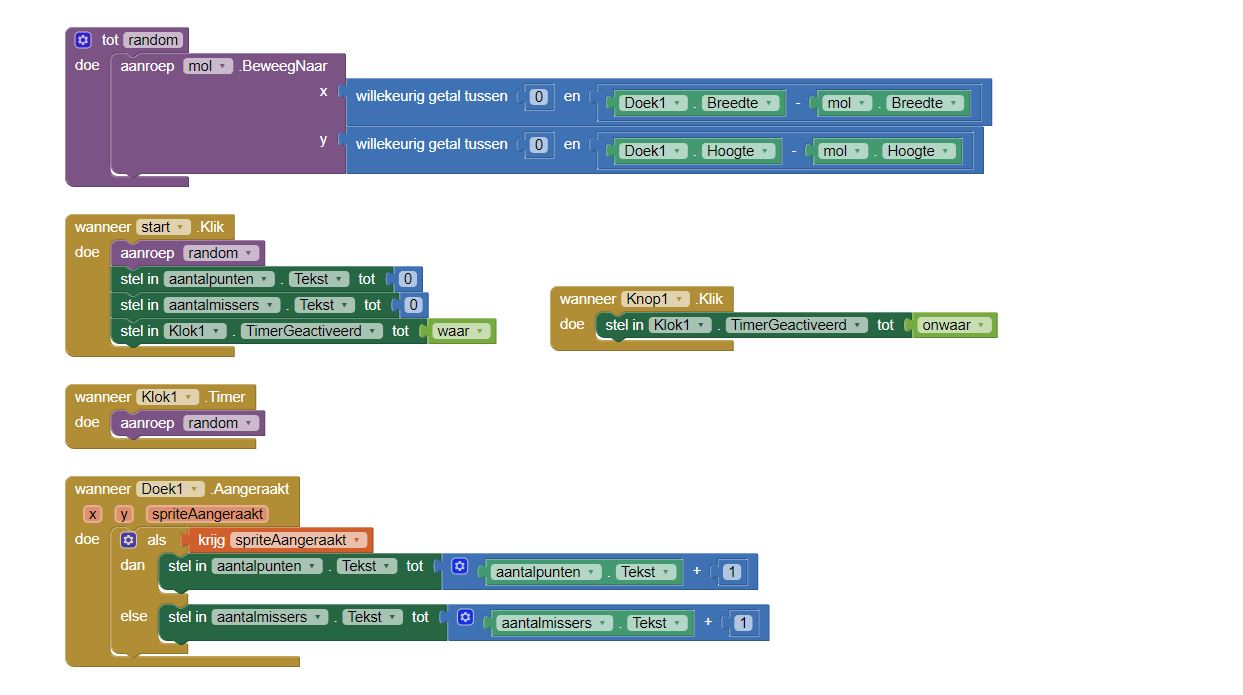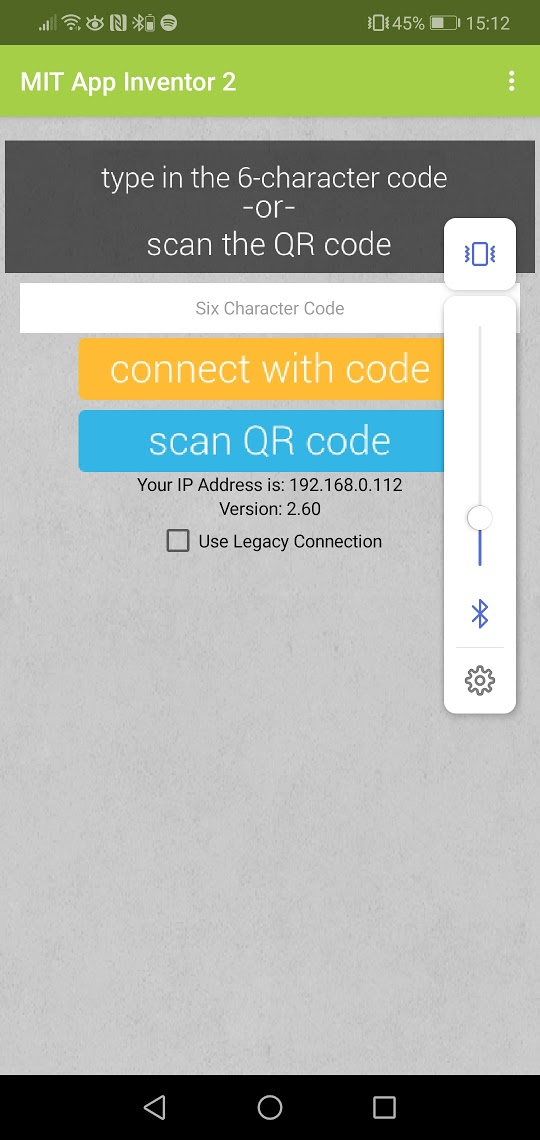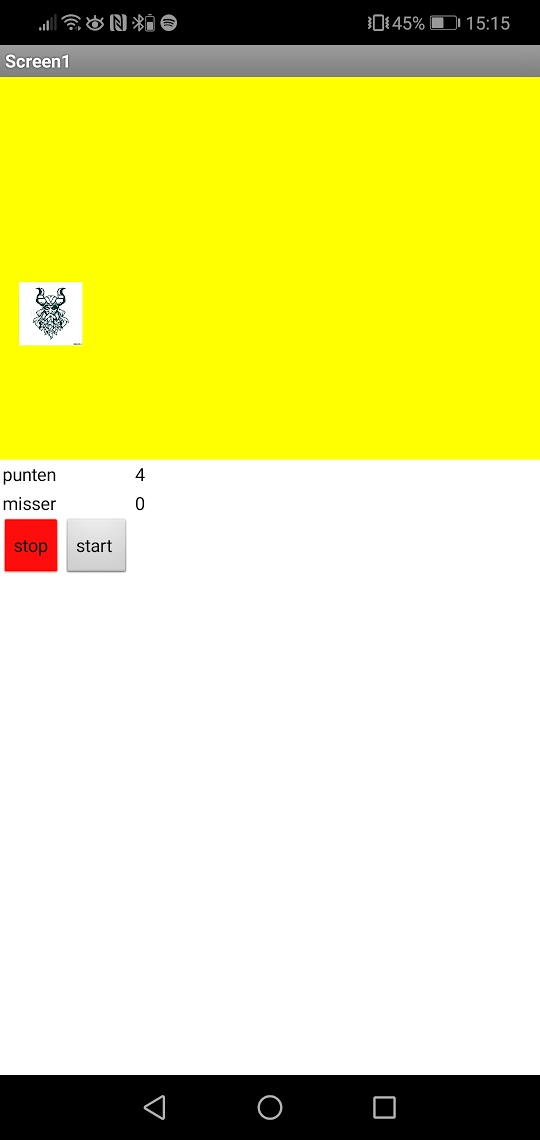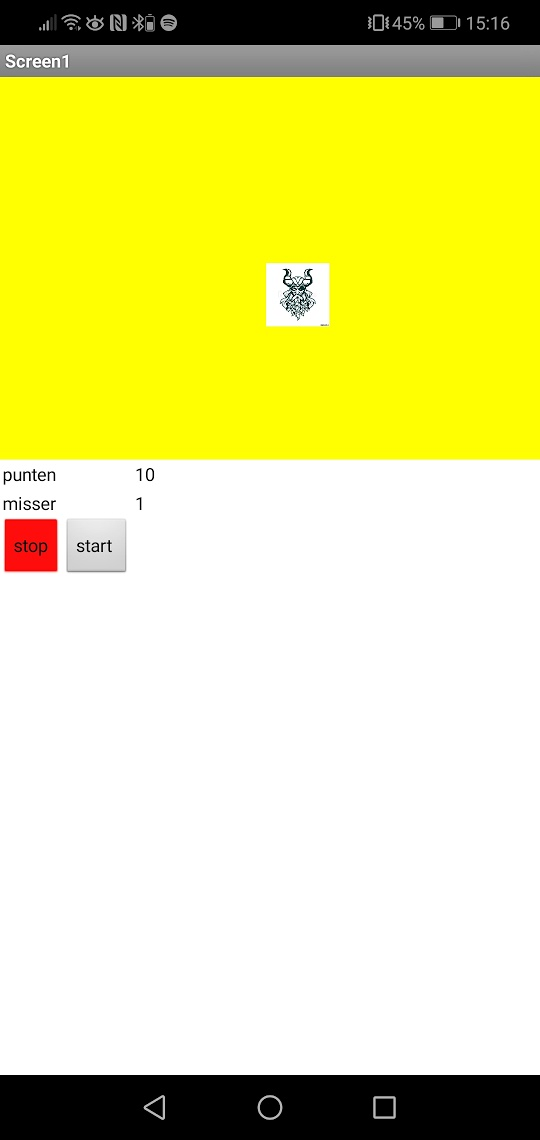### link the the group assigment

interface

Last update: July 10, 2021